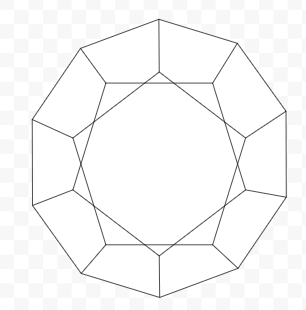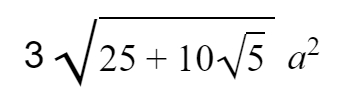# Program for Surface area of Dodecahedron in C++

## What is Dodecahedron?

The word ‘dodecahedron’ is derived from the Greek words where, dodeca means ‘twelve’ and hedron specifies ‘faces’. Dodecahedron in geometric is a 3-D platonic or regular solid with twelve flat faces. Like, other figures dodecahedron also have properties and those are −

• 20 polyhedron vertices
• 30 polyhedron edges
• 12 pentagonal faces, as a pentagon is a five-sided polygon

Given below is the figure of dodecahedron## Problem

Given with an edge, the program must find the surface area of dodecahedron where surface area is the total space occupied by the faces of the given figure.

To calculate surface area of dodecahedron there is a formula −## Example

Input-: side=5
Output-: 516.143

## ALGORITHM

Start
Step 1 -> declare function to find area of dodecahedron
double area(int side)
return ((3 * sqrt(25 + 10 * (sqrt(5)))) * (pow(side, 2)))
Step 2 -> In main()
Declare variable int side=5
Print area(side)
Stop

## CODE

#include <bits/stdc++.h>
using namespace std;
//function to find area of dodecahedron
double area(int side){
return ((3 * sqrt(25 + 10 * (sqrt(5)))) * (pow(side, 2))) ;
}
int main(){
int side = 5;
cout<< "Surface area of dodecahedron is : " << area(side);
return 0;
}

## Output

Surface area of dodecahedron is : 516.143